#### You may also like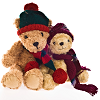### Teddy Town

There are nine teddies in Teddy Town - three red, three blue and three yellow. There are also nine houses, three of each colour. Can you put them on the map of Teddy Town according to the rules?### Four Triangles Puzzle

Cut four triangles from a square as shown in the picture. How many different shapes can you make by fitting the four triangles back together?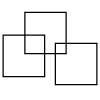### Three Squares

What is the greatest number of squares you can make by overlapping three squares?

# Rod Ratios

##### Age 7 to 11Challenge Level

Here are three pairs of rods: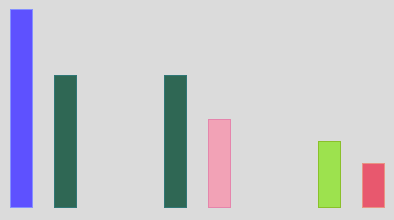The ratio of each of these pairs is 3 : 2.

Can you use the interactivity below to show that this is true?

What is the ratio of the pair below? Again, you could use the interactivity to show your thinking.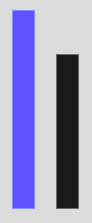Here is another pair of rods: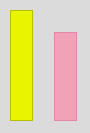Can you use the interactivity to find other pairs of single rods with the same ratio as the yellow to the pink?

Again, using only pairs of single rods what pairs can you find with the same ratio as those below?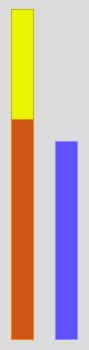Using only pairs of single rods what pairs can you find with the ratio 9 : 6?